Main Content

# firceqrip

Constrained equiripple FIR filter

## Syntax

```B = firceqrip(n,Fo,DEV) B = firceqrip(...,'slope',r) B = firceqrip('minorder',[Fp Fst],DEV) B = firceqrip(...,'passedge') B = firceqrip(...,'stopedge') B = firceqrip(...,'high') B = firceqrip(...,'min') B = firceqrip(...,'invsinc',C) B = firceqrip(...,'invdiric',C) ```

## Description

`B = firceqrip(n,Fo,DEV)` designs an order `n` filter (filter length equal `n` + 1) lowpass FIR filter with linear phase.

`firceqrip` produces the same equiripple lowpass filters that `firpm` produces using the Parks-McClellan algorithm. The difference is how you specify the filter characteristics for the function.

The input argument `Fo` specifies the frequency at the upper edge of the passband in normalized frequency (0<`Fo`<1). The two-element vector `dev` specifies the peak or maximum error allowed in the passband and stopbands. Enter ```[d1 d2]``` for `dev` where `d1` sets the passband error and `d2` sets the stopband error.

`B = firceqrip(...,'slope',r)` uses the input keyword '`slope`' and input argument `r` to design a filter with a nonequiripple stopband. `r` is specified as a positive constant and determines the slope of the stopband attenuation in dB/normalized frequency. Greater values of `r` result in increased stopband attenuation in dB/normalized frequency.

`B = firceqrip('minorder',[Fp Fst],DEV)` designs filter with the minimum number of coefficients required to meet the deviations in `DEV` = [d1 d2] while having a transition width no greater than `Fst``Fp`, the difference between the stopband and passband edge frequencies. You can specify `'mineven'` or `'minodd'` instead of `'minorder'` to design minimum even order (odd length) or minimum odd order (even length) filters, respectively. The `'minorder'` option does not apply when you specify the `'min'` (minimum-phase), `'invsinc'`, or the `'invdiric'` options.

`B = firceqrip(...,'passedge')` designs a filter where `Fo` specifies the frequency at which the passband starts to rolloff.

`B = firceqrip(...,'stopedge')` designs a filter where `Fo` specifies the frequency at which the stopband begins.

`B = firceqrip(...,'high')` designs a high pass FIR filter instead of a lowpass filter.

`B = firceqrip(...,'min')` designs a minimum-phase filter.

`B = firceqrip(...,'invsinc',C)` designs a lowpass filter whose magnitude response has the shape of an inverse sinc function. This may be used to compensate for sinc-like responses in the frequency domain such as the effect of the zero-order hold in a D/A converter. The amount of compensation in the passband is controlled by `C`, which is specified as a scalar or two-element vector. The elements of `C` are specified as follows:

• If `C` is supplied as a real-valued scalar or the first element of a two-element vector, `firceqrip` constructs a filter with a magnitude response of 1/sinc(`C`*`pi`*`F`) where `F` is the normalized frequency.

• If `C` is supplied as a two-element vector, the inverse-sinc shaped magnitude response is raised to the positive power `C(2)`. If we set `P=C(2)`, `firceqrip` constructs a filter with a magnitude response 1/sinc(`C`*`pi`*`F`)P.

If this FIR filter is used with a cascaded integrator-comb (CIC) filter, setting `C(2)` equal to the number of stages compensates for the multiplicative effect of the successive sinc-like responses of the CIC filters.

Note

Since the value of the inverse sinc function becomes unbounded at `C=1/F`, the value of `C` should be greater the reciprocal of the passband edge frequency. This can be expressed as `Fo<1/C`. For users familiar with CIC decimators, `C` is equal to 1/2 the product of the differential delay and decimation factor.

`B = firceqrip(...,'invdiric',C)` designs a lowpass filter with a passband that has the shape of an inverse Dirichlet sinc function. The frequency response of the inverse Dirichlet sinc function is given by $\left\{rC{\left(\frac{\mathrm{sin}\left(f/2r\right)}{\mathrm{sin}\left(Cf/2\right)}\right\}}^{p}$where C, r, and p are scalars. The input `C` can be a scalar or vector containing 2 or 3 elements. If `C` is a scalar, `p` and `r` equal 1. If `C` is a two-element vector, the first element is `C` and the second element is `p`, `[C p]`. If `C` is a three-element vector, the third element is `r`, ```[C p r]```.

## Examples

To introduce a few of the variations on FIR filters that you design with `firceqrip`, these five examples cover both the default syntax `b = firceqrip(n,wo,del)` and some of the optional input arguments. For each example, the input arguments `n`, `wo`, and `del` remain the same.

### Filter design using `firceqrip`

Design a 30th order FIR filter using `firceqrip`.

`b = firceqrip(30,0.4,[0.05 0.03]); fvtool(b)`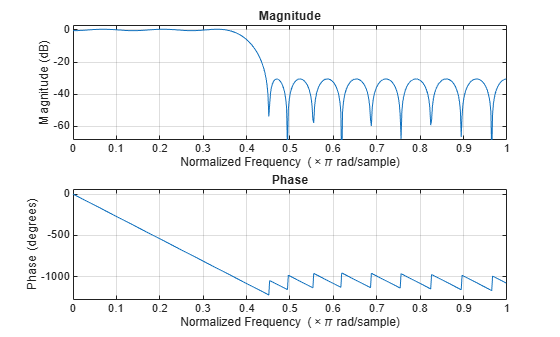Design a minimum order FIR filter using `firceqrip`. The passband edge and stopband edge frequencies are 0.35$\pi$ and 0.45$\pi$ rad/sample. The allowed deviations are 0.02 and 1e-4.

`b = firceqrip('minorder',[0.35 0.45],[0.02 1e-4]); fvtool(b)`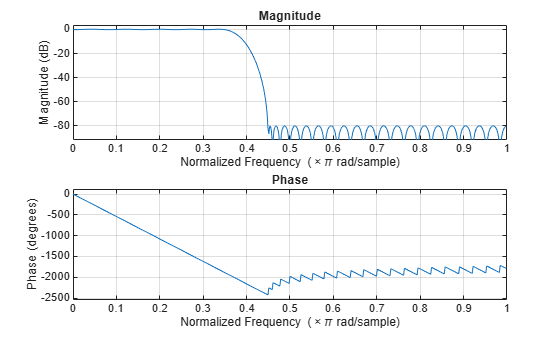Design a 30th order FIR filter with the `stopedge` keyword to define the response at the edge of the filter stopband.

`b = firceqrip(30,0.4,[0.05 0.03],'stopedge'); fvtool(b)`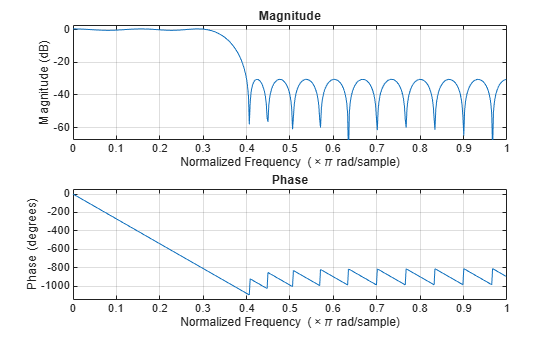Design a 30th order FIR filter with the `slope` keyword and r = 20.

`b = firceqrip(30,0.4,[0.05 0.03],'slope',20,'stopedge'); fvtool(b)`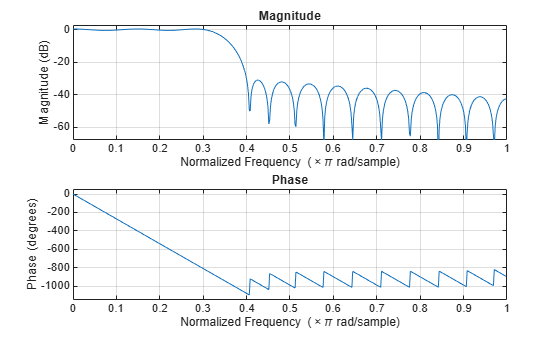Design a 30th order FIR filter defining the stopband and specifying that the resulting filter is minimum phase with the `min` keyword.

`b = firceqrip(30,0.4,[0.05 0.03],'stopedge','min'); fvtool(b)`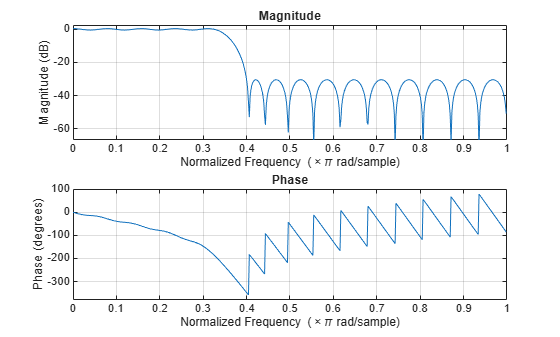Comparing this filter to the filter in Figure 1. The cutoff frequency `wo = 0.4` now applies to the edge of the stopband rather than the point at which the frequency response magnitude is 0.5.

Viewing the zero-pole plot shown here reveals this is a minimum phase FIR filter - the zeros lie on or inside the unit circle, z = 1

`fvtool(b,'polezero')`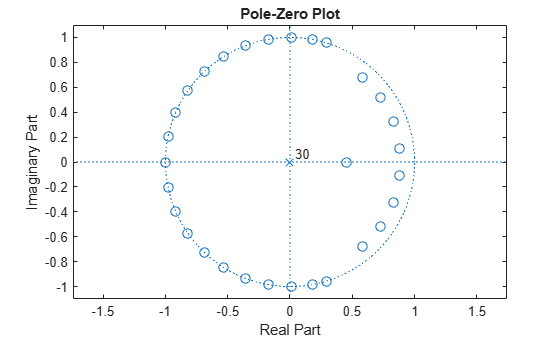Design a 30th order FIR filter with the `invsinc` keyword to shape the filter passband with an inverse sinc function.

`b = firceqrip(30,0.4,[0.05 0.03],'invsinc',[2 1.5]); fvtool(b)`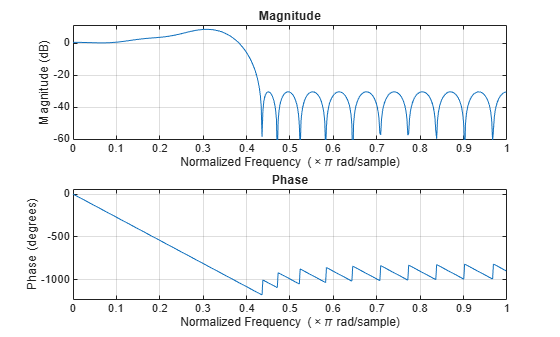The inverse sinc function being applied is defined as 1/sinc(2*w)^1.5.

### Inverse-Dirichlet-Sinc-Shaped Passband

Design two order 30 constrained equiripple FIR filters with inverse-Dirichlet-sinc-shaped passbands. The cutoff frequency in both designs is pi/4 radians/sample. Set `C=1` in one design `C=2` in the second design. The maximum passband and stopband ripple is 0.05. Set `p=1` in one design and `p=2` in the second design.

Design the filters.

```b1 = firceqrip(30,0.25,[0.05 0.05],'invdiric',[1 1]); b2 = firceqrip(30,0.25,[0.05 0.05],'invdiric',[2 2]);```

Obtain the filter frequency responses using `freqz`. Plot the magnitude responses.

``` [h1,~] = freqz(b1,1); [h2,w] = freqz(b2,1); plot(w,abs(h1)); hold on; plot(w,abs(h2),'r'); axis([0 pi 0 1.5]); xlabel('Radians/sample'); ylabel('Magnitude'); legend('C=1 p=1','C=2 p=2');```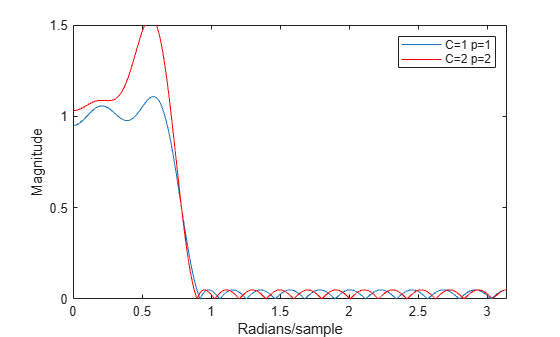Inspect the stopband ripple in the design with `C=1` and `p=1`. The constrained design sets the maximum ripple to be 0.05. Zoom in on the stopband from the cutoff frequency of pi/4 radians/sample to 3pi/4 radians/sample.

``` figure; plot(w,abs(h1)); set(gca,'xlim',[pi/4 3*pi/4]); grid on;```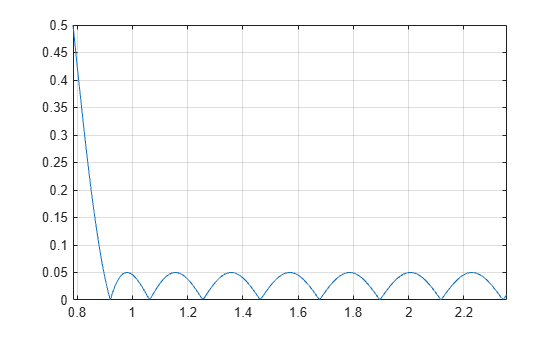## See Also

Introduced in R2011a

Watch now